# Piecewise Functions

## A Function Can be in Pieces

We can create functions that behave differently based on the input (x) value.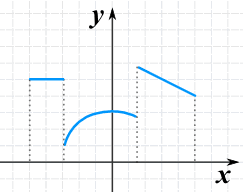A function made up of 3 pieces

### Example:

• when x is less than 2, it gives x2,
• when x is exactly 2 it gives 6
• when x is more than 2 and less than or equal to 6 it gives the line 10-x

It looks like this: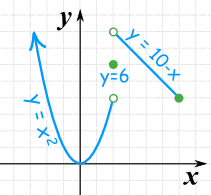(a solid dot means "including",
an open dot means "not including")

And this is how we write it: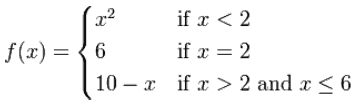The Domain (all the values that can go into the function) is all Real Numbers up to and including 6, which we can write like this:

Dom(f) = (-∞, 6] (using Interval Notation)

Dom(f) = {x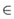| x ≤ 6} (using Set Builder Notation)

And here are some example values:

X Y
−4 16
−2 4
0 0
1 1
2 6
3 7

### Example: Here is another piecewise function:which looks like: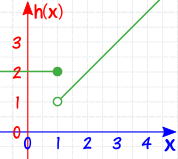What is h(−1)?

x is ≤ 1, so we use h(x) = 2, so h(−1) = 2

What is h(1)?

x is ≤ 1, so we use h(x) = 2, so h(1) = 2

What is h(4)?

x is > 1, so we use h(x) = x, so h(4) = 4

Piecewise functions let us make functions that do anything we want!

### Example: A Doctor's fee is based on the length of time.

• Up to 6 minutes costs \$50
• Over 6 and up to 15 minutes costs \$80
• Over 15 minutes costs \$80 plus \$5 per minute above 15 minutes

Which we can write like this:You visit for 12 minutes, what is the fee? \$80

You visit for 20 minutes, what is the fee? \$80+\$5(20-15) = \$105

## The Absolute Value Function

The Absolute Value Function is a famous Piecewise Function.

It has two pieces:

• below zero: -x
• from 0 onwards: xf(x) = |x|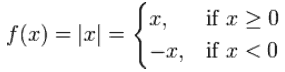## The Floor Function

The Floor Function is a very special piecewise function. It has an infinite number of pieces:The Floor Function